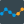#### Versions

You are viewing the RapidMiner Studio documentation for version 9.6 - Check here for latest version

#Holt-Winters (Time Series)

## Synopsis

This operator trains an Holt-Winters forecasting model with triple exponential smoothing.

## Description

The Holt-Winters forecasting method applies a triple exponential smoothing for level, trend and seasonal components.

A Holt-Winters model is defined by its three order parameters, alpha, beta, gamma. Alpha specifies the coefficient for the level smoothing. Beta specifies the coefficient for the trend smoothing. Gamma specifies the coefficient for the seasonal smoothing. There is also a parameter for the type of seasonality: Additive seasonality, where each season changes by a constant number. Multiplicative seasonality, where each season changes by a factor.

This operator works only on numerical time series.

## Differentiation

This operator is similar to other modeling operators, but is specifically designed to work on time series data. One of the implications of this is, that the forecast model should be applied on the same data it was trained on.

###Apply Forecast

This operator receives a trained Forecast Model (e.g. the Holt-Winters model) and creates the forecast for the time series it was trained on.

###ARIMA

This operator trains an ARIMA model (Autoregressive Integrated Moving Average) on time series data to perform a forecast.

###Default Forecast

This operator trains a Default Forecast model (predicting single value) on time series data to perform a forecast.

###Function and Seasonal Component Forecast

This operator trains a Function and Seasonal Forecast model (combining fitted function and seasonal component values) on time series data to perform a forecast.

## Input

•example set (IOObject)

The ExampleSet which contains the time series data as an attribute.

## Output

•forecast model (IOObject)

The Holt-Winters model (forecast model) fitted to the specified time series attribute. It also contains the original time series values.

•original (IOObject)

The ExampleSet that was given as input is passed through without changes.

## Parameters

• time_series_attribute

The time series attribute (numerical) for which the Holt-Winters model should be build. The required attribute can be selected from this option. The attribute name can be selected from the drop down box of the parameter if the meta data is known.

Range:
• has_indices

This parameter indicates if there is an index attribute associated with the time series. If this parameter is set to true, the index attribute has to be selected.

Range:
• indices_attribute

If the parameter has indices is set to true, this parameter defines the associated index attribute. It can be either a date, date_time or numeric value type attribute. The attribute name can be selected from the drop down box of the parameter if the meta data is known.

Range:
• alpha:_coefficient_for_level_smoothing

The parameter alpha specifies the coefficient for the level smoothing.

Range:
• beta:_coefficient_for_trend_smoothing

The parameter beta specifies the coefficient for the trend smoothing.

Range:
• gamma:_coefficient_for_seasonality_smoothing

The parameter gamma specifies the coefficient for the seasonal smoothing.

Range:
• period:_length_of_one_period

This parameter specifies the length of one seasonal period in the data. For weekly data this is often 7, for monthly data 12. The period has to be smaller or equal to half of the length of the time series.

Range:
• seasonality_model

This parameter specifies the type of seasonality.

• additive: Additive seasonality, where each season changes by a constant number.
• multiplicative: Multiplicative seasonality, where each season changes by a factor.
Range:

## Tutorial Processes

### Holt-Winters on monthly milk production

This tutorial process shows the basic usage of the Holt-Winters operator, by training a Holt-Winters model on the Monthly Milk Production data.